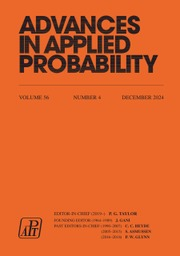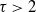Home
Hostname: page-component-cf9d5c678-xvx2z Total loading time: 0.206 Render date: 2021-07-31T20:04:44.823Z Has data issue: true Feature Flags: { "shouldUseShareProductTool": true, "shouldUseHypothesis": true, "isUnsiloEnabled": true, "metricsAbstractViews": false, "figures": true, "newCiteModal": false, "newCitedByModal": true, "newEcommerce": true, "newUsageEvents": true }Advances in Applied Probability

# Subgraphs in preferential attachment models

Published online by Cambridge University Press:  03 September 2019

## Abstract

We consider subgraph counts in general preferential attachment models with power-law degree exponent$\tau > 2$ . For all subgraphs H, we find the scaling of the expected number of subgraphs as a power of the number of vertices. We prove our results on the expected number of subgraphs by defining an optimization problem that finds the optimal subgraph structure in terms of the indices of the vertices that together span it and by using the representation of the preferential attachment model as a Pólya urn model.

## MSC classification

Type
Original Article
Information
Advances in Applied Probability , September 2019 , pp. 898 - 926

## Access options

Get access to the full version of this content by using one of the access options below. (Log in options will check for institutional or personal access. Content may require purchase if you do not have access.)

## References

Albert, R. and Barabási, A.-L. (2002). Statistical mechanics of complex networks. Rev. Modern Phys. 74, 4797.CrossRefGoogle Scholar
Barabási, A.-L. and Albert, R. (1999). Emergence of scaling in random networks. Science 286, 509512.CrossRefGoogle ScholarPubMed
Berger, N., Borgs, C., Chayes, J. T. and Saberi, A. (2014). Asymptotic behavior and distributional limits of preferential attachment graphs. Ann. Prob. 42, 140.CrossRefGoogle Scholar
Boguñá, M. and Pastor-Satorras, R. (2003). Class of correlated random networks with hidden variables. Phys. Rev. E 68, 036112, 13pp.CrossRefGoogle ScholarPubMed
Bollobás, B. (1980). A probabilistic proof of an asymptotic formula for the number of labelled regular graphs. Europ. J. Combinatorics 1, 311316.CrossRefGoogle Scholar
Bollobás, B. and Riordan, O. M. (2002). Mathematical Results on Scale-Free Random Graphs. Wiley–WCH, Weinheim.CrossRefGoogle Scholar
Bollobás, B., Riordan, O., Spencer, J. and Tusnády, G. (2001). The degree sequence of a scale-free random graph process. Random Structures Algorithms 18, 279290.CrossRefGoogle Scholar
Britton, T., Deijfen, M. and Martin-Löf, A. (2006). Generating simple random graphs with prescribed degree distribution. J. Statist. Phys. 124, 13771397.CrossRefGoogle Scholar
Chung, F. and Lu, L. (2002). The average distances in random graphs with given expected degrees. Proc. Nat. Acad. Sci. USA 99, 1587915882.CrossRefGoogle ScholarPubMed
Dommers, S., van der Hofstad, R. and Hooghiemstra, G. (2010). Diameters in preferential attachment models. J. Statist. Phys. 139, 72107.CrossRefGoogle Scholar
Eggemann, N. and Noble, S. D. (2011). The clustering coefficient of a scale-free random graph. Discrete Appl. Math. 159, 953965.CrossRefGoogle Scholar
Faloutsos, M., Faloutsos, P. and Faloutsos, C. (1999). On power-law relationships of the internet topology. Comput. Commun. Rev. 29, 251262.CrossRefGoogle Scholar
Gao, C. and Lafferty, J. (2017). Testing network structure using relations between small subgraph probabilities. Preprint. Available at https://arxiv.org/abs/1704.06742v1.Google Scholar
Garavaglia, A. (2019). Preferential attachment models for dynamic networks. Doctoral Thesis, Eindhoven University of Techonolgy.Google Scholar
Jordan, J. (2006). The degree sequences and spectra of scale-free random graphs. Random Structures Algorithms 29, 226242.CrossRefGoogle Scholar
Krioukov, D. (2010). Hyperbolic geometry of complex networks. Phys. Rev. E 82, 036106, 18pp.CrossRefGoogle ScholarPubMed
Marcus, D. and Shavitt, Y. (2012). RAGE – a rapid graphlet enumerator for large networks. Comput. Networks 56, 810819.CrossRefGoogle Scholar
Maugis, P.-A. G., Priebe, C. E., Olhede, S. C. and Wolfe, P. J. (2019). Statistical inference for network samples using subgraph counts. Preprint. Available at https://arxiv.org/abs/1701.00505v3.Google Scholar
Milo, R. (2002). Network motifs: simple building blocks of complex networks. Science 298, 824827.CrossRefGoogle ScholarPubMed
Milo, R. (2004). Superfamilies of evolved and designed networks. Science 303, 15381542.CrossRefGoogle ScholarPubMed
Onnela, J.-P., Saramäki, J., Kertész, J. and Kaski, K. (2005). Intensity and coherence of motifs in weighted complex networks. Phys. Rev. E 71, 065103, 4pp.CrossRefGoogle ScholarPubMed
Prokhorenkova, L. O. (2017). General results on preferential attachment and clustering coefficient. Optimization Lett. 11, 279298.CrossRefGoogle Scholar
Prokhorenkova, L. A. and Krot, A. V (2016). Local clustering coefficients in preferential attachment models. Dokl. Math. 94, 623626.CrossRefGoogle Scholar
Prokhorenkova, L., Ryabchenko, A. and Samosvat, E. (2013). Generalized preferential attachment: tunable power-law degree distribution and clustering coefficient. In Algorithms and Models for the Web Graph, eds Bonato, A., Mitzenmacher, M. and Praat, P., Springer, Cham, pp. 185202.Google Scholar
Shen-Orr, S. S., Milo, R., Mangan, S. and Alon, U. (2002). Network motifs in the transcriptional regulation network of Escherichia coli. Nature Genet. 31, 6468.CrossRefGoogle ScholarPubMed
Szymaski, J. (2005). Concentration of vertex degrees in a scale-free random graph process. Random Structures Algorithms 26, 224236.CrossRefGoogle Scholar
van der Hofstad, R. (2019+). Random Graphs and Complex Networks, Vol. 2. In preparation.Google Scholar
van der Hofstad, R. (2017). Random Graphs and Complex Networks, Vol. 1. Cambridge University Press.CrossRefGoogle Scholar
van der Hofstad, R., van Leeuwaarden, J. S. H. and Stegehuis, C. (2017). Optimal subgraph structures in scale-free configuration models. Preprint. Available at https://arxiv.org/abs/1709.03466v1.Google Scholar
Vázquez, A., Pastor-Satorras, R. and Vespignani, A. (2002). Large-scale topological and dynamical properties of the Internet. Phys. Rev. E 65, 066130, 12pp.CrossRefGoogle ScholarPubMed
Wernicke, S. and Rasche, F. (2006). FANMOD: a tool for fast network motif detection. Bioinformatics 22, 11521153.CrossRefGoogle ScholarPubMed
Wuchty, S., Oltvai, Z. N. and Barabási, A.-L. (2003). Evolutionary conservation of motif constituents in the yeast protein interaction network. Nature Genet. 35, 176179.CrossRefGoogle ScholarPubMed
2
Cited by

# Send article to Kindle

Note you can select to send to either the @free.kindle.com or @kindle.com variations. ‘@free.kindle.com’ emails are free but can only be sent to your device when it is connected to wi-fi. ‘@kindle.com’ emails can be delivered even when you are not connected to wi-fi, but note that service fees apply.

Find out more about the Kindle Personal Document Service.

Subgraphs in preferential attachment models
Available formats
×

# Send article to Dropbox

To send this article to your Dropbox account, please select one or more formats and confirm that you agree to abide by our usage policies. If this is the first time you use this feature, you will be asked to authorise Cambridge Core to connect with your <service> account. Find out more about sending content to Dropbox.

Subgraphs in preferential attachment models
Available formats
×

# Send article to Google Drive

To send this article to your Google Drive account, please select one or more formats and confirm that you agree to abide by our usage policies. If this is the first time you use this feature, you will be asked to authorise Cambridge Core to connect with your <service> account. Find out more about sending content to Google Drive.

Subgraphs in preferential attachment models
Available formats
×
×